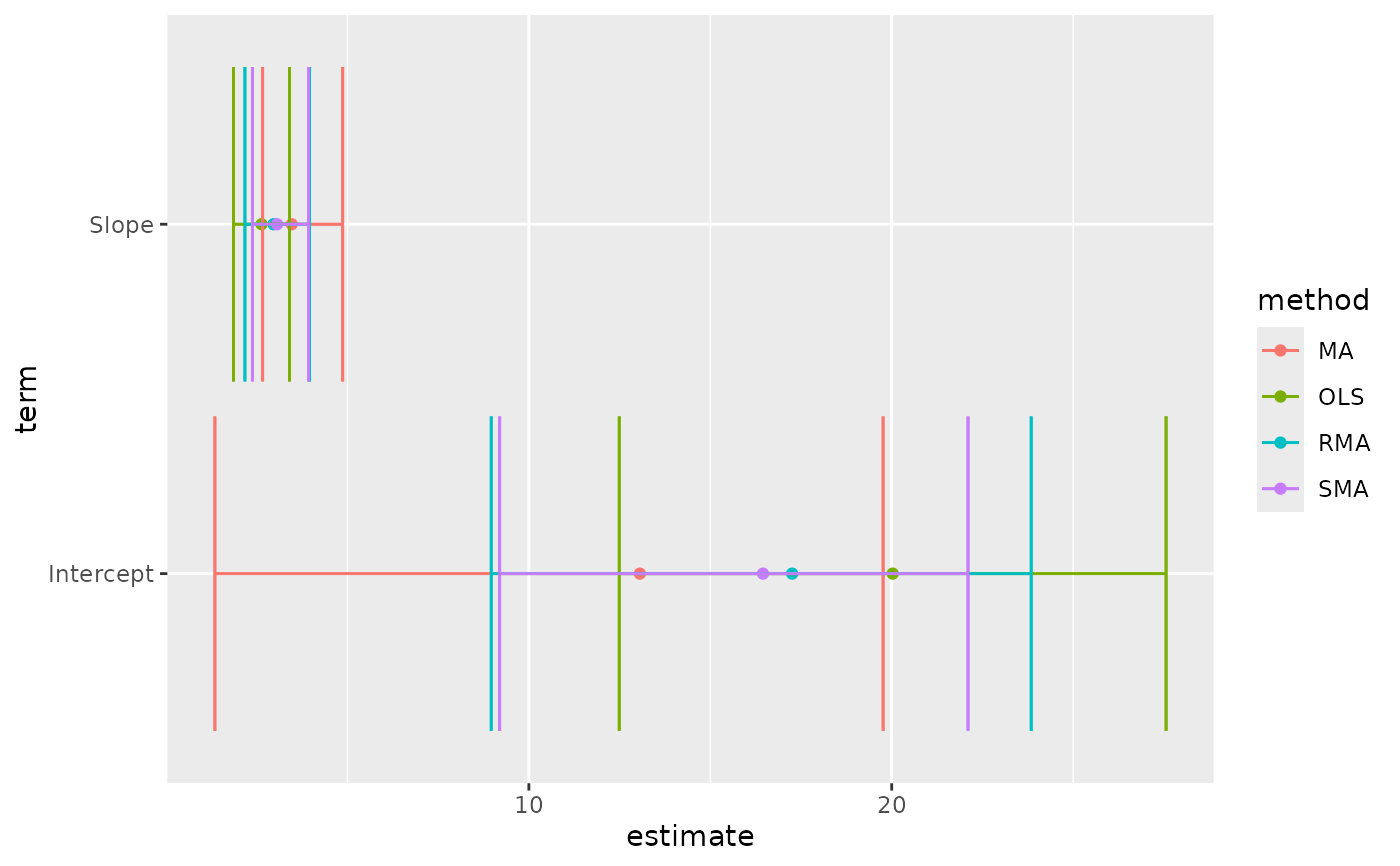Glance accepts a model object and returns a tibble::tibble() with exactly one row of model summaries. The summaries are typically goodness of fit measures, p-values for hypothesis tests on residuals, or model convergence information.

Glance never returns information from the original call to the modelling function. This includes the name of the modelling function or any arguments passed to the modelling function.

Glance does not calculate summary measures. Rather, it farms out these computations to appropriate methods and gathers the results together. Sometimes a goodness of fit measure will be undefined. In these cases the measure will be reported as NA.

# S3 method for lmodel2
glance(x, ...)

## Arguments

x A lmodel2 object returned by lmodel2::lmodel2(). Additional arguments. Not used. Needed to match generic signature only. Cautionary note: Misspelled arguments will be absorbed in ..., where they will be ignored. If the misspelled argument has a default value, the default value will be used. For example, if you pass conf.lvel = 0.9, all computation will proceed using conf.level = 0.95. Additionally, if you pass newdata = my_tibble to an augment() method that does not accept a newdata argument, it will use the default value for the data argument.

glance(), lmodel2::lmodel2()

Other lmodel2 tidiers: tidy.lmodel2()

## Value

A tibble::tibble() with exactly one row and columns:

nobs

Number of observations used.

p.value

P-value corresponding to the test statistic.

r.squared

R squared statistic, or the percent of variation explained by the model. Also known as the coefficient of determination.

theta

Angle between OLS lines lm(y ~ x) and lm(x ~ y)

H

H statistic for computing confidence interval of major axis slope

## Examples


library(lmodel2)

data(mod2ex2)
Ex2.res <- lmodel2(Prey ~ Predators, data=mod2ex2, "relative", "relative", 99)
Ex2.res#>
#> Model II regression
#>
#> Call: lmodel2(formula = Prey ~ Predators, data = mod2ex2, range.y =
#> "relative", range.x = "relative", nperm = 99)
#>
#> n = 20   r = 0.8600787   r-square = 0.7397354
#> Parametric P-values:   2-tailed = 1.161748e-06    1-tailed = 5.808741e-07
#> Angle between the two OLS regression lines = 5.106227 degrees
#>
#> Permutation tests of OLS, MA, RMA slopes: 1-tailed, tail corresponding to sign
#> A permutation test of r is equivalent to a permutation test of the OLS slope
#> P-perm for SMA = NA because the SMA slope cannot be tested
#>
#> Regression results
#>   Method Intercept    Slope Angle (degrees) P-perm (1-tailed)
#> 1    OLS  20.02675 2.631527        69.19283              0.01
#> 2     MA  13.05968 3.465907        73.90584              0.01
#> 3    SMA  16.45205 3.059635        71.90073                NA
#> 4    RMA  17.25651 2.963292        71.35239              0.01
#>
#> Confidence intervals
#>   Method 2.5%-Intercept 97.5%-Intercept 2.5%-Slope 97.5%-Slope
#> 1    OLS      12.490993        27.56251   1.858578    3.404476
#> 2     MA       1.347422        19.76310   2.663101    4.868572
#> 3    SMA       9.195287        22.10353   2.382810    3.928708
#> 4    RMA       8.962997        23.84493   2.174260    3.956527
#>
#> Eigenvalues: 269.8212 6.418234
#>
#> H statistic used for computing C.I. of MA: 0.006120651
#>
tidy(Ex2.res)#> # A tibble: 8 x 6
#>   method term      estimate conf.low conf.high p.value
#>   <fct>  <chr>        <dbl>    <dbl>     <dbl>   <dbl>
#> 1 MA     Intercept    13.1      1.35     19.8     0.01
#> 2 MA     Slope         3.47     2.66      4.87    0.01
#> 3 OLS    Intercept    20.0     12.5      27.6     0.01
#> 4 OLS    Slope         2.63     1.86      3.40    0.01
#> 5 RMA    Intercept    17.3      8.96     23.8     0.01
#> 6 RMA    Slope         2.96     2.17      3.96    0.01
#> 7 SMA    Intercept    16.5      9.20     22.1    NA
#> 8 SMA    Slope         3.06     2.38      3.93   NA   glance(Ex2.res)#> # A tibble: 1 x 5
#>   r.squared theta    p.value       H  nobs
#>       <dbl> <dbl>      <dbl>   <dbl> <int>
#> 1     0.740  5.11 0.00000116 0.00612    20
# this allows coefficient plots with ggplot2
library(ggplot2)
ggplot(tidy(Ex2.res), aes(estimate, term, color = method)) +
geom_point() +
geom_errorbarh(aes(xmin = conf.low, xmax = conf.high)) +
geom_errorbarh(aes(xmin = conf.low, xmax = conf.high))